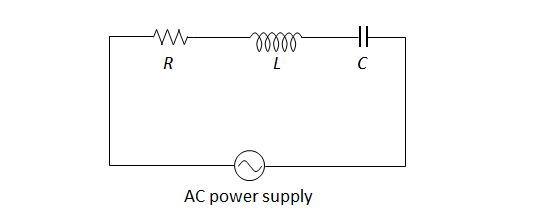# Frequency of AC powerThe above is an RLC series circuit, where the resistance of the resistor, the self-inductance of the inductor and the capacitance of the capacitor are $R$, $L$ and $C$, respectively. If the impedance of the circuit is $R$, then what is the frequency of the AC power supply?

×